# Inter maths solurions for quadratic expressions

Intermediate second year mathematics IIA quadratic equations text book exercise 3(b) solutions are given.

These solutions are very easy to understand.

Study the text book. Observe the solutions and try them in your own methods

You can see the solutions for maths IIA inter

Complex numbers solutions for textbook

De Moivre’s Theorem textbook solutions

Exercise 3(b)

Exercise 3(c)

You can see solutions for Inter maths IIB

1. Circle

3. Parabola

4. Ellipse

You can see the solutions for first year maths 1A and 1B for examination purpose

Inter maths 1A solutions

3. Matrices

You can also see the solutions for 1b

1. Locus

The straight line sa

Straight lines la

## Exercise 3(b) chapter 3 second year inter maths IIA

Exercise 3(b)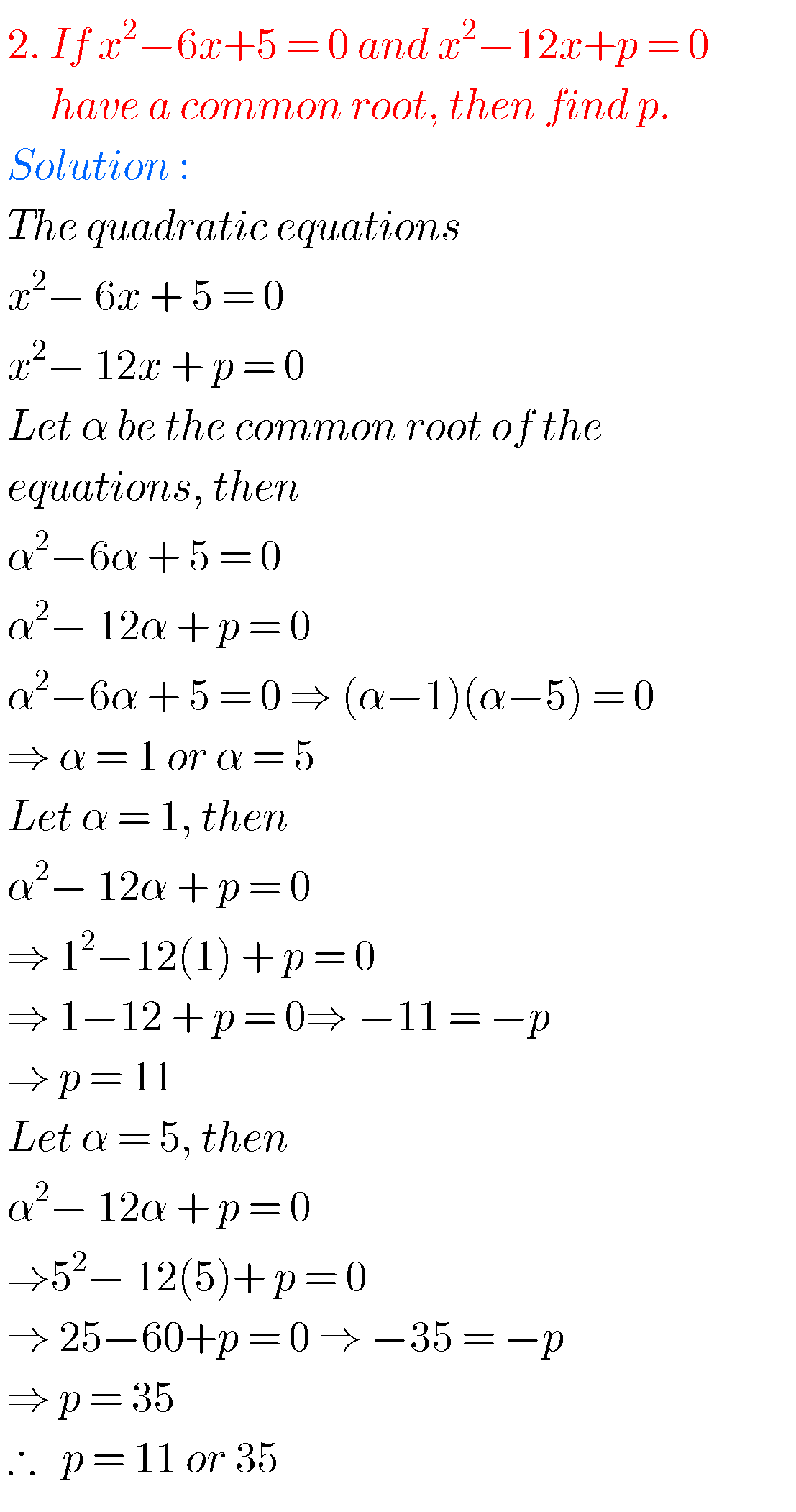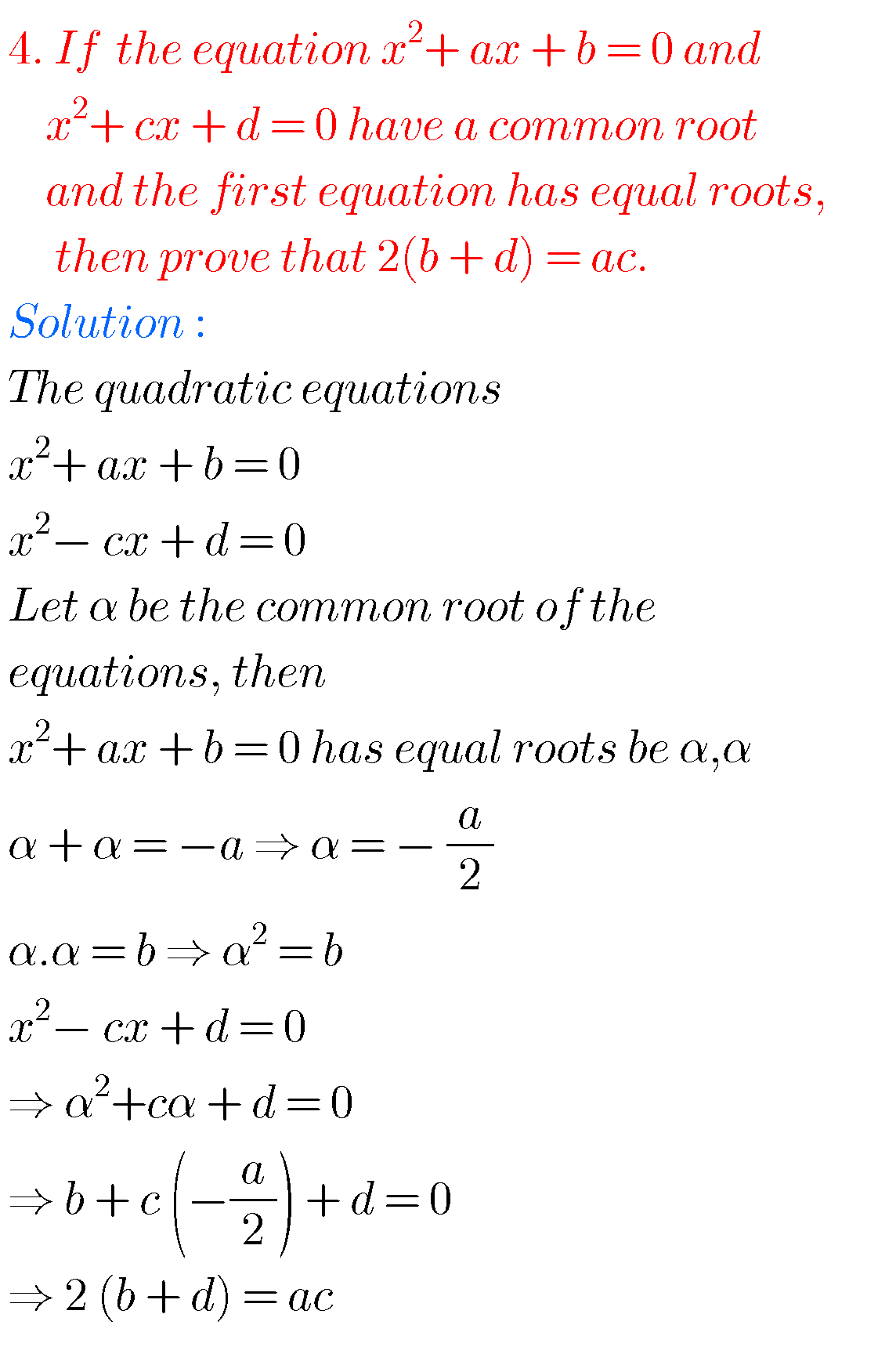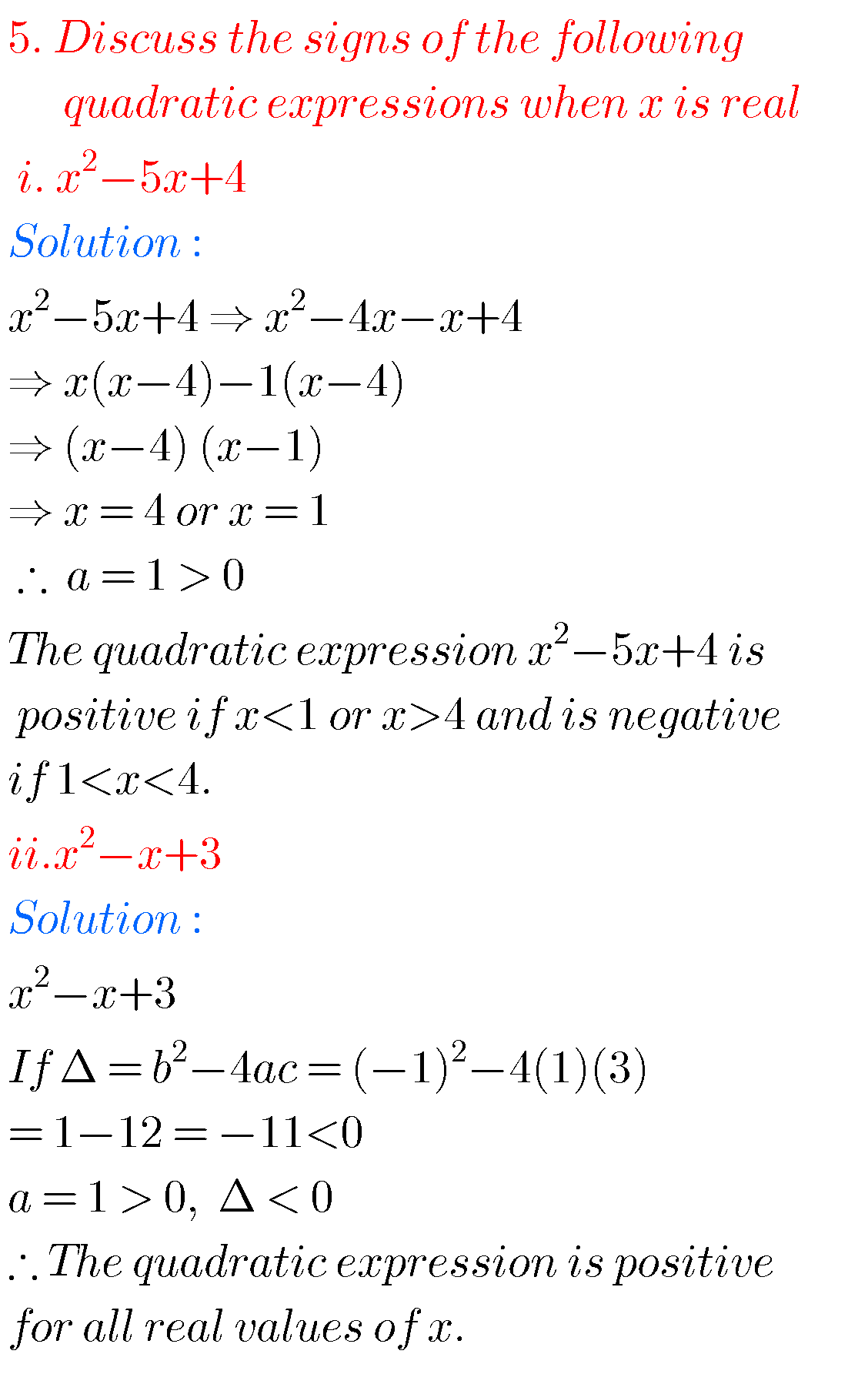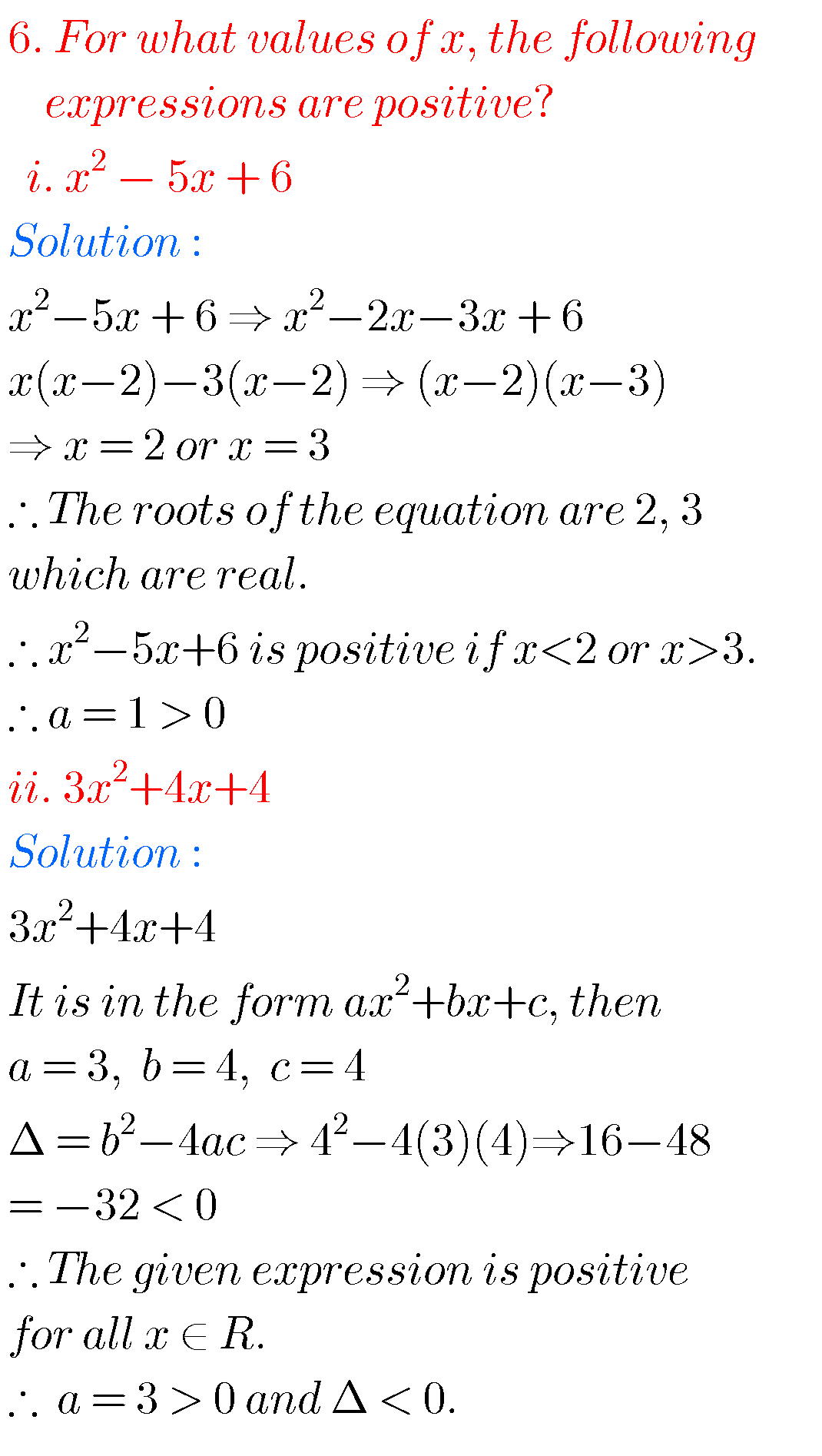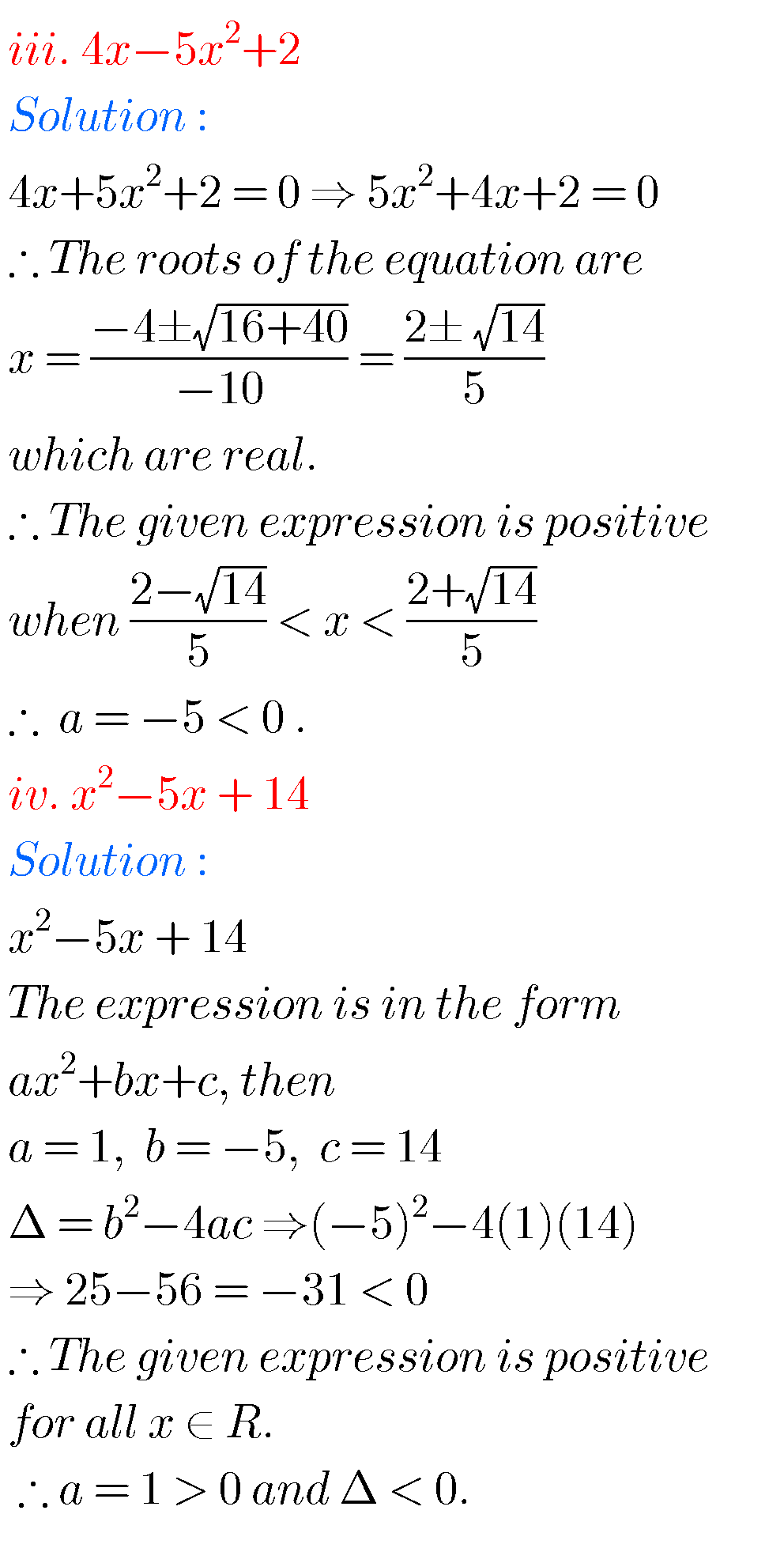###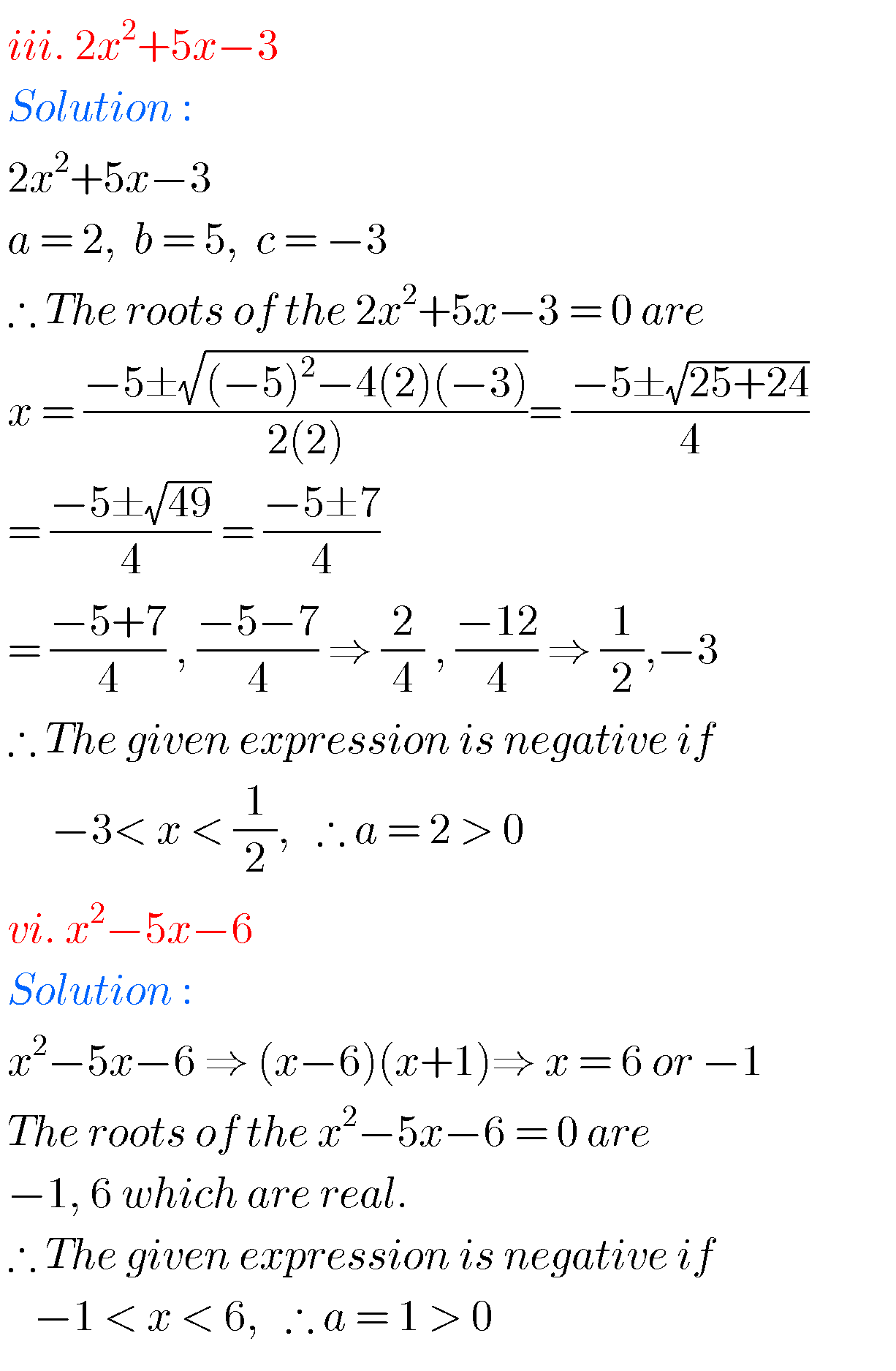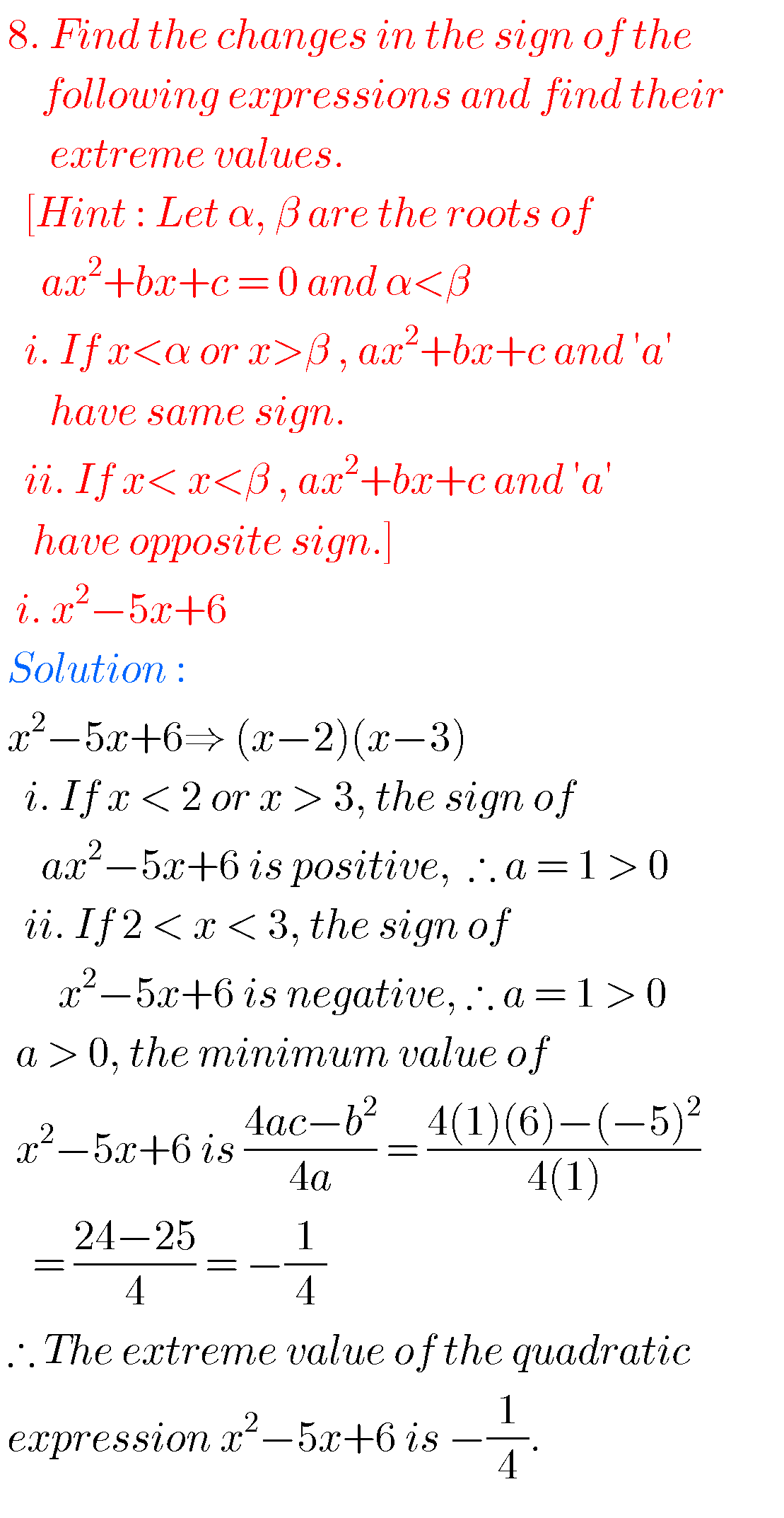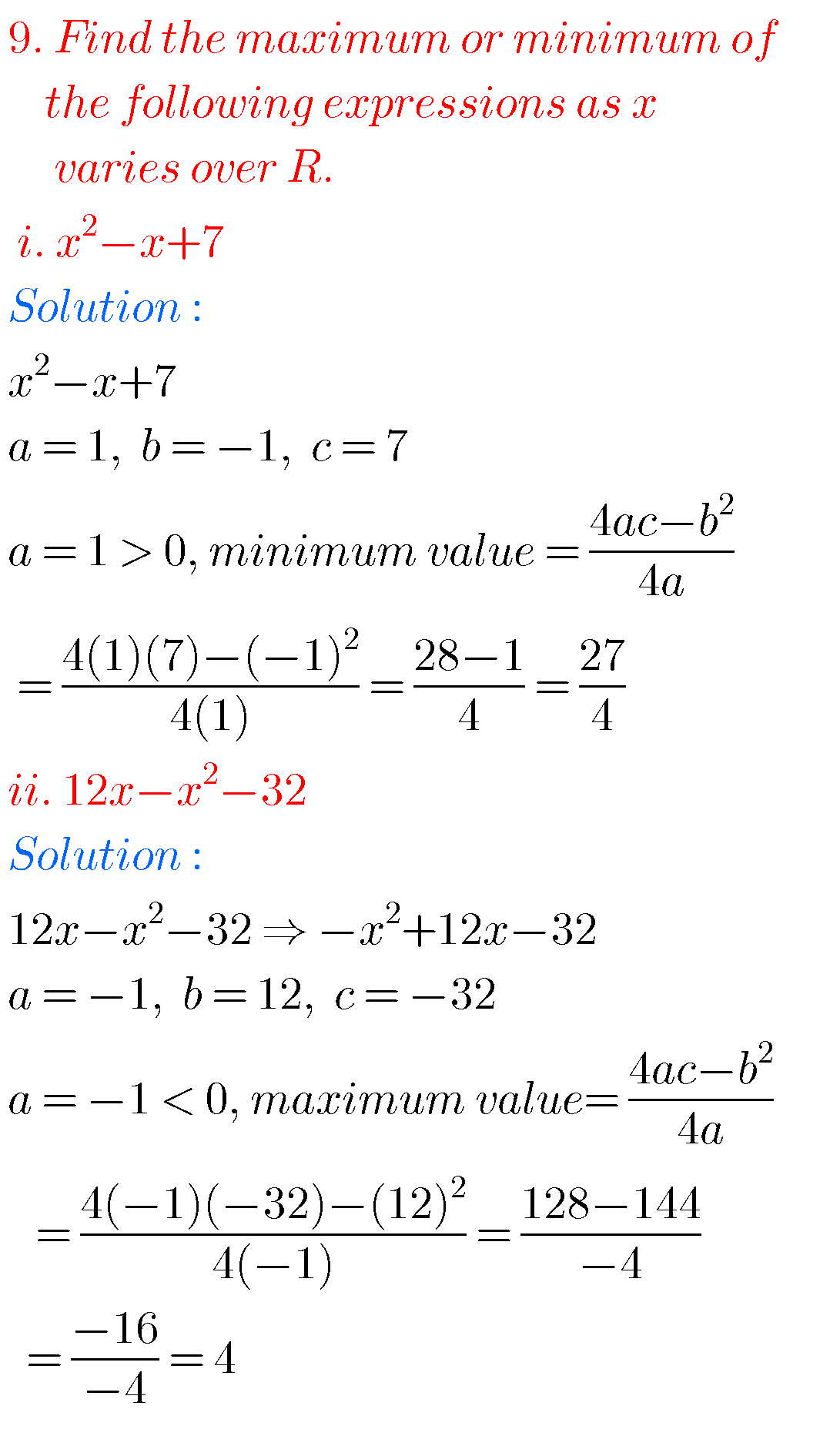### Quadratic expressions ( text book) exercise 3(b) solutionsNote : Observe the solutions and try them in your own methods.

Inter 1a exercise 6(a) solutions

class 10 maths polynomials solutions

Ncert maths class 8 exponents and powers

Ncert maths class 7 fractions and decimals

Ncert maths class 6 playing with numbers

1.2.